The NIST reference dataset contains 27 datasets for validating nonlinear regression analysis software. The datasets were distributed by level of difficulty: 8 lower, 11 average and 8 higher. Here, will be presented the LAB Fit results for the average level. For each dataset a function is indicated and the initial values are suggested (two groups). The two groups were tested by LAB Fit. For most of the datasets, the uncertainty could be given with 1 or 2 significant digits. However, the LAB Fit results were presented with 3 digits for the uncertainty. The value 1 was assumed for the "POWER" parameter and for the tolerance was assumed the default value: 1.0E-06. Although LAB Fit calculates the covariance matrix (for error propagation purposes, confidence and prediction bands), the results won't be presented because the SRD / NIST doesn't certify it.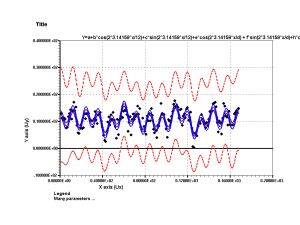Confidence and prediction bands.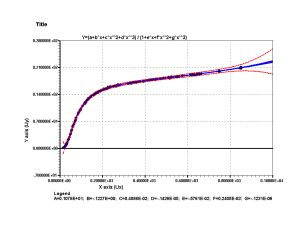Confidence and prediction bands and extrapolation.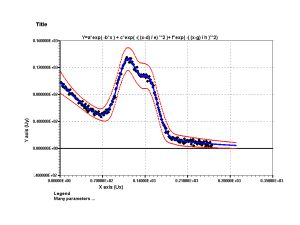Confidence and prediction bands and extrapolation.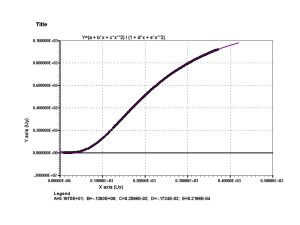Extrapolation.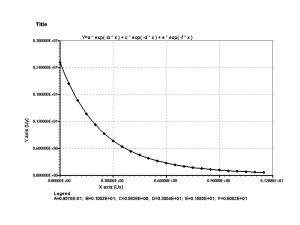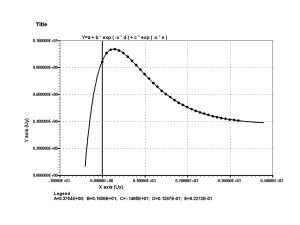Extrapolation.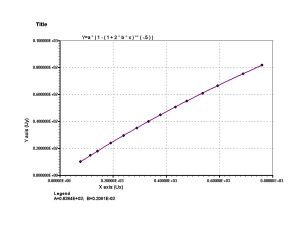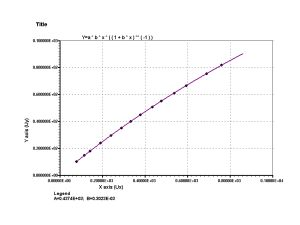Extrapolation.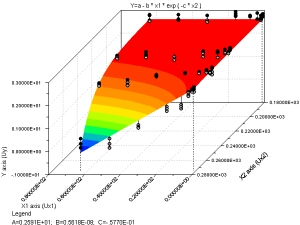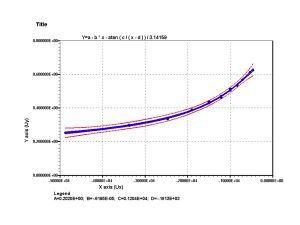Confidence and prediction bands and extrapolation. ENSO - Dataset, initial and certified values                                           y = a + b*cos(2*3.14159*x/12) + c*sin(2*3.14159*x/12) + e*cos(2*3.14159*x/d) + f*sin(2*3.14159*x/d) + h*cos(2*3.14159*x/g) + i*sin(2*3.14159*x/g) a = 10.511 Ý 0.175   b = 3.076 Ý 0.243   c = 0.533 Ý 0.244 d = 44.314 Ý 0.944   e = -1.623 Ý 0.281   f = 0.526 Ý 0.481 g = 26.891 Ý 0.416   h = 0.215 Ý 0.515   i = 1.496 Ý 0.255 Observation:     the results agree with the validated values by the SRD of the NIST (initial values suggested: primary and secondary groups). HAHN1 - Dataset, initial and certified values y = (a+b*x+c*x**2+d*x**3) / (1+e*x+f*x**2+g*x**3) a = 1.078 Ý 0.171   b = -0.1227 Ý 0.0120 c = 0.004086 Ý 0.000225   d = -1.426E-06 Ý 0.276E-06 e = -0.005761 Ý 0.000247   f = 2.405E-04 Ý 0.104E-04 g = -1.231E-07 Ý 0.130E-07 Observation:     the results agree with the validated values by the SRD of the NIST (initial values suggested: primary and secondary groups). GAUSS3 - Dataset, initial and certified values y = a*exp( -b*x ) + c*exp( -( (x-d) / e) **2 ) + f*exp( -( (x-g) / h )**2) a = 98.940 Ý 0.530   b = 0.010946 Ý 0.000126 c = 100.696 Ý 0.813   d = 111.636 Ý 0.353 e = 23.300 Ý 0.366   f = 73.71 Ý 1.21 g = 147.762 Ý 0.405   h = 19.668 Ý 0.378 Observation:     the results agree with the validated values by the SRD of the NIST (initial values suggested: primary and secondary groups). KIRBY2 - Dataset, initial and certified values y = (a + b*x + c*x**2) / (1 + d*x + e*x**2) a = 1.6745 Ý 0.0880   b = -0.13928 Ý 0.00412 c = 25.961E-04 Ý 0.419E-04   d = -17.242E-04 Ý 0.589E-04 e = 21.665E-06 Ý 0.201E-06 Observation:     the results agree with the validated values by the SRD of the NIST (initial values suggested: primary and secondary groups). LANCZOS1 - Dataset, initial and certified values y = a * exp( -b * x ) + c * exp( -d * x ) + e * exp( -f * x ) a = 951000000.274E-10 Ý 0.533E-10   b = 1000000000.128E-09 Ý 0.275E-09 c = 860700000.134E-09 Ý 0.136E-09   d = 3000000000.235E-09 Ý 0.333E-09 e = 1557599999.838E-09 Ý 0.188E-09   f = 5000000000.112E-09 Ý 0.111E-09 Observation:     the results agree with the validated values by the SRD of the NIST (initial values suggested: primary and secondary groups;   tolerance = 1.0E-13). LANCZOS2 - Dataset, initial and certified values y = a * exp( -b * x ) + c * exp( -d * x ) + e * exp( -f * x ) a = 0.096251 Ý 0.000668   b = 1.00573 Ý 0.00340 c = 0.86425 Ý 0.00172   d = 3.00783 Ý 0.00417 e = 1.55290 Ý 0.00237   f = 5.00288 Ý 0.00140 Observation:     the results agree with the validated values by the SRD of the NIST (initial values suggested: primary and secondary groups). MGH17 - Dataset, initial and certified values y = a + b * exp ( -x * d ) + c * exp ( -x * e ) a = 0.37541 Ý 0.00207   b = 1.936 Ý 0.220 c = -1.465 Ý 0.222   d = 0.012867 Ý 0.000449 e = 0.022123 Ý 0.000895 Observation:     the results agree with the validated values by the SRD of the NIST (initial values suggested: primary and secondary groups). MISRA1C - Dataset, initial and certified values y = a * ( 1 - ( 1 + 2 * b * x ) ** ( -.5 ) ) a = 636.43 Ý 4.66 b = 20.814E-05 Ý 0.177E-05 Observation:     the results agree with the validated values by the SRD of the NIST (initial values suggested: primary and secondary groups). MISRA1D - Dataset, initial and certified values y = a * b * x * ( ( 1 + b * x ) ** ( -1 ) ) a = 437.37 Ý 3.65 b = 30.227E-05 Ý 0.293E-05 Observation:     the results agree with the validated values by the SRD of the NIST (initial values suggested: primary and secondary groups). NELSON - Dataset, initial and certified values Attention: y = logY, being Y the original values. y = a - b * x1 * exp ( -c * x2 ) a = 2.5907 Ý 0.0192 b = 0.562E-08 Ý 0.611E-08 c = -0.05770 Ý 0.00396 Observation:     the results agree with the validated values by the SRD of the NIST (initial values suggested: primary and secondary groups). ROSZMAN1 - Dataset, initial and certified values y = a - b * x - atan ( c / ( x - d ) ) / 3.14159 a = 0.2020 Ý 0.0192 b = -0.620E-05 Ý 0.321E-05 c = 1204.5 Ý 74.1 d = -181.3 Ý 49.6 Observation:     the results agree with the validated values by the SRD of the NIST (initial values suggested: primary and secondary groups). Top All the LAB Fit results are statistically correct...                                   For all the initial values...

| Back |